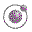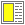Expressions, Types, and Functions - slide 6 : 46

Examples of expressions and their values
• Let us assume that x has the value 3

• Simple expressions

• 7    has the value 7

• (+ x 5)    has the value 8

• Conditional expressions

• (if (even? x) 7 (+ x 5))    has the value 8

• Lambda expressions

• (lambda (x) (+ x 1))    has the value 'the function that adds one to its parameter'

• HTML mirror expressions

• ```(htmlLAML and HTML mirror functions